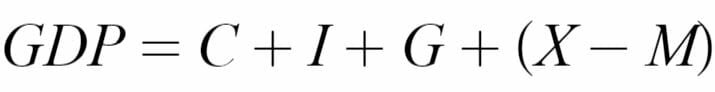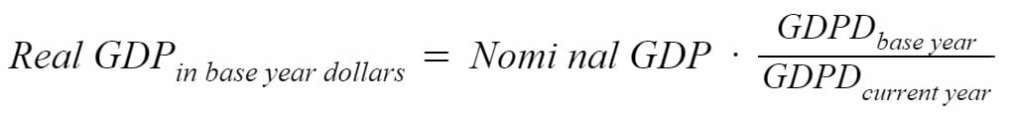# Nominal GDP vs. Real GDP

How inflation can paint a misleading picture

## What is Nominal vs. Real Gross Domestic Product (GDP)?

Nominal Gross Domestic Product (GDP) and Real GDP both quantify the total value of all goods produced in a country in a year. However, real GDP is adjusted for inflation, while nominal GDP isn’t. Thus, real GDP is almost always slightly lower than its equivalent nominal figure. In most circumstances, the real GDP (and real GDP per capita) shows a more accurate picture of a country’s economic performance since it can be more easily compared to past figures. Thus, we can deduce whether a country really is better or worse off year over year.### How is Nominal GDP Calculated?

Nominal GDP is calculated using the following equation:Where:

C – Private consumption

– Gross investment

G – Government investment

X – Exports

– Imports

For example, if a country reports \$5 trillion in private consumption, \$10 trillion in gross investment, \$4 trillion in government investment, exported \$2 trillion of goods and imported \$1 trillion, its nominal GDP would be:The GDP would be \$20 trillion.

### How is Real GDP Calculated?

To calculate real GDP, we must discount the nominal GDP by a GDP deflator. The GDP deflator is a measure of the price levels of new goods that are available in a country’s domestic market. It includes prices for businesses, the government, and private consumers. The GDP deflator essentially removes inflation from the equation and enables us to compare the GDP of a recent year to the GDP of a target (or “base”) year.

Different price indices such as the consumer price index could theoretically also be used in the calculation of GDP. However, CPI only considers prices for consumer goods and thus ignores a substantial part of the economy. Thus, the GDP deflator is the preferred measure. The equation for calculating real GDP is:Where:

GDPD – GDP Deflator

Let’s say that in 2018, the nominal GDP of a country was \$8 trillion. Using the year 2000 as the base year (i.e., with a value of 100), the 2018 GDP deflator returns a value of 140. Therefore, we can convert from nominal to real:Thus, the real GDP would be \$7.1 trillion. We can then compare the resulting amount to the nominal GDP in the year 2000 to draw insights about the economy’s performance relative to expectations over the given time period.

### More Resources

CFI offers the Financial Modeling & Valuation Analyst (FMVA)™ certification program for those looking to take their careers to the next level. To learn more about related topics, check out the following CFI resources:

• Price Elasticity
• Utility Theory
• Supply and Demand
• Economic Exposure

### Financial Analyst Certification

Become a certified Financial Modeling and Valuation Analyst (FMVA)® by completing CFI’s online financial modeling classes!